6th grade (11y) + least common multiple (LCM) - examples

1. CherriesCherries in the bowl can be divided equally among 19 or 13 or 28 children. How many is the minimum cherries in the bowl?
2. PlumsIn the bowl are plums. How many would be there if we can divide it equally among 8, 10 and 11 children?
3. PlumberPlumber had to cut the metal strip with dimensions 380 cm and 60 cm to the largest squares so that no waste. Calculate the length of the sides of a square. How many squares cut it?
4. LCMWhat is the least common multiple of 36, 44, 21?
5. Game roomWinner can took win in three types of jettons with value 3, 30 and 100 dollars. What is minimal value of win payable in this values of jettons?
6. GroupGroup of kids wanted to ride. When the children were divided into groups of 3 children 1 remain. When divided into groups of 4 children 1 remain. When divided into groups of 6 children 1 missed. After divided to groups of 5 children its OK. How many are t
7. FractionsSort fractions ? by its size. Result write as three serial numbers 1,2,3.
8. School booksAt the beginning of the school year, the teacher gave out 480 books and 220 textbooks. How many students were in the class?
9. Around the flowerbed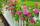Around a rectangular flowerbed with dimensions 5.25 m and 3.5 m are to be planted roses equally spaced so that the roses found in every corner of the flowerbed and consumed them as little as possible. a) At what distance are planted roses? b) How many ros
10. CubesCarol with cut bar 12 cm x 12 cm x 135 cm to the cubes. Find the sum of all the surfaces of the resulting cubes.
11. Square tilesThe room has dimensions 12 meters and 5.6 meters. Determine the number of square tiles and their largest dimension to exactly cover the floor.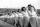Practitioners lined up in rectangle with row with four, five or six exercisers, one always missing to full rectangle. How many exercisers were on the field, if they have estimated not been more than 100?
13. Unknown integerFind the smallest integer that: divided by 2, the remainder is 1 divided by 3 the remainder is 2, divided by 4 remainder is 3, ... divided by eight reminder is 7, by 9 reminder is 8.
14. Bouquet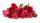Gardener tying bouquet of flowers for 8 and none was left. Then he found that he could tying bouquet of 6 flowers and also none was left. How many have gardener flowers (minimum and maximum) if they had between 50 and 100 flowers?
15. Square roomWhat is the size of the smallest square room, which can pave with tiles with dimensions 55 cm and 45 cm? How many such tiles is needed?
16. Apples and pearsMom divided 24 apples and 15 pears to children. Each child received the same number of apples and pears - same number as his siblings. How many apples (j=?) and pears (h=?) received each child?
17. BusesBuses Ikarus and Karosa simultaneously started at 8:00 from the final station. Ikarus is returned to the station after 30 minutes. Karosa after 45 minutes. At what time both buses again returned to the station?
18. Buses 4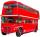intervals: 1st bus 40 min. 2nd bus 2h 3rd bud 20min How long take them to meet - as soon as possible?
19. Parachutists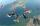Parachutists during freefall firstly held in groups of 4, then of 6, then 9, 12 and finally of 18 members. How many parachutists jump at least should be, if at each group must all be involved.
20. Fewer than 500 sheep,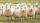There are fewer than 500 sheep, but if they stand in a double, triple, quadruple, five and sixth order, one sheep will remain. But they can stand in the seventh order. How many are sheep?

Do you have an interesting mathematical example that you can't solve it? Enter it, and we can try to solve it.

To this e-mail address, we will reply solution; solved examples are also published here. Please enter e-mail correctly and check whether you don't have a full mailbox.

Do you want to calculate least common multiple two or more numbers?